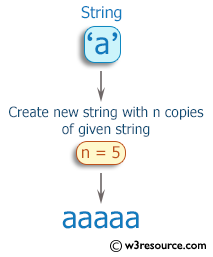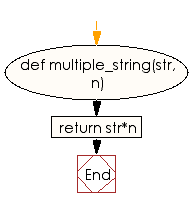﻿ Ruby Basic exercises: Create a new string which is n copies of a given string where n is a non-negative integer - w3resource# Ruby Basic Exercises: Create a new string which is n copies of a given string where n is a non-negative integer

## Ruby Basic: Exercise-3 with Solution

Write a Ruby program to create a new string which is n copies of a given string where n is a non-negative integer.Ruby Code:

``````def multiple_string(str, n)
return str*n
end
print multiple_string('a', 1),"\n"
print multiple_string('a', 2),"\n"
print multiple_string('a', 3),"\n"
print multiple_string('a', 4),"\n"
print multiple_string('a', 5),"\n"
``````

Output:

```a
aa
aaa
aaaa
aaaaa
```

Flowchart:Ruby Code Editor: